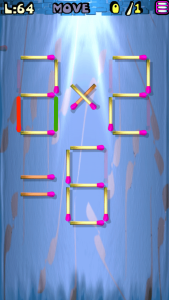# Move the Matches Puzzles 61-80 Walkthrough

Walkthrough for all the solutions and answers to the game Move the Matches Puzzles by K-Lab. Get the app on the Google Play Store to play along if you do not already have it.

### Level 61Instructions: Remove 2 matches to get 3 triangles, all equal

### Level 62Instructions: Remove 1 match to correct the equation

Solution: 1+4=5

### Level 63Instructions: Add 4 matches to get 4 squares

### Level 64Instructions: Move 1 match to correct the equation

Solution: 3+2=6

### Level 65Instructions: Move 1 match to get 3 squares

### Level 66Instructions: Remove 2 matches to correct the equation

Solution: 4-1=3

### Level 67Instructions: Move 2 matches to correct the equation

Solution: 2×3=6

### Level 68Instructions: Remove 2 matches to get 3 squares

### Level 69Instructions: Move 2 matches to get 3 squares

### Level 70Instructions: Remove 5 matches to get 3 squares

### Level 71Instructions: Remove 1 match to correct the equation

Solution: 2+7=9

### Level 72Instructions: Move 1 match to get 3 triangles, all equal

### Level 73Instructions: Move 2 matches to get 2 squares

### Level 74Instructions: Move 3 matches to get 2 triangles

### Level 75Instructions: Remove 2 matches to correct the equation

Solution: 1+1=2

### Level 76Instructions: Remove 1 match to correct the equation

Solution: 5-1=4

### Level 77Instructions: Move 1 match to correct the equation

Solution: 3+8=11

### Level 78Instructions: Remove 1 match to correct the equation

Solution: 9+5=14

### Level 79Instructions: Remove 9 matches to get 4 squares

### Level 80Instructions: Add 3 matches to get 3 triangles